6. ELECTROMAGNETIC INDUCTION

Q.1. What are the advantages or application of eddy currents ?

Ans ⇒  Following are the advantages of eddy currents :
(i) Diathermy : These are used for deep heat treatment i.e., localised heating of tissues in human body. This treatment is called Diathermy.

(ii) Concept of eddy currents is used in energy metres to record the consumption of electricity.

(iii) Speedometer : The eddy currents are produced in the aluminium drum in which a magnet attached to the axle of the wheel rotates according to the speed of the vehicle. The pointer attached to the drum is deflected in the direction of rotation of the drum.

(iv) Electromagnetic brakes : These are used in trains. The large eddy currents are produced in the metallic drum which oppose the motion of the drum.

(v) Dead beat galvanometer : Electromagnetic damping is used to stop coils in a shorter interval of time. When the coil wound over a metallic frame is deflected, eddy currents are produced in the metallic frame which oppose the motion of the coil.

(vi) Induction Furnace makes use of heating effect of eddy currents.

(vii) Induction motor or a.c. motor also works by eddy currents.

Q.2. What are eddy currents ? How are they produced ? Give two important application of eddy currents.

Ans ⇒  Eddy currents are the currents induced in the body of a conductor.
When the magnetic flux linked with a conductor changes, eddy currents are produced.
Two important applications of eddy currents are in electromagnetic damping and induction motor.

Q.3. Define the term ‘self-inductance’. Give its unit. Write an expression for the energy stored in an inductor when a steady current ‘I’ is passed through it. Is this energy electric or magnetic ?

Ans ⇒  Self inductance of a coil is defined as the emf induced in the coil when the current passing through it changes at the unit rate. Its SI unit is Henry (H) when a current I flows through an inductor of inductance L, the energy stored in it is
U = 1/2 LI2
The energy is stored as magnetic energy.

Q.4. Derive an expression for the mutual inductance of a pair of coaxial solenoids having number of turns N1 and N2.

Ans ⇒  Consider two solenoids S1 and S2 with S1 closely would over S2. Let I be the length and A the common cross-section area of each solenoid.
Magnetic field B at any point inside S1 due to current I1 in it is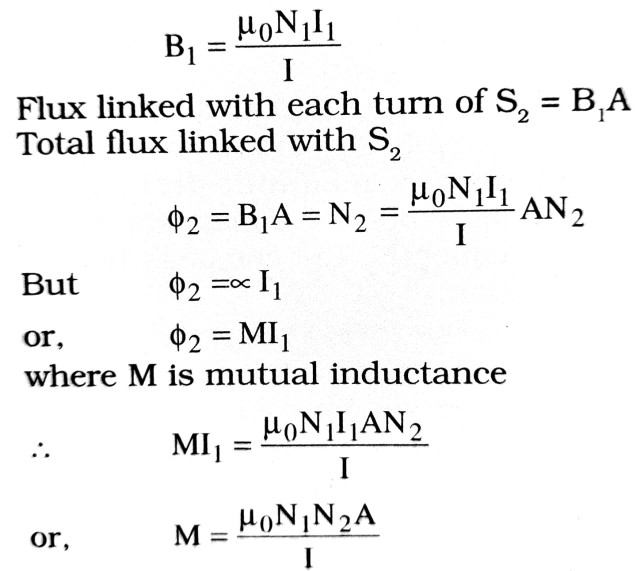Q.5. In what sense are eddy currents considered undesirable in a transformer and how are these reduced in such a device ?

Ans ⇒  In transformer, considerable amount of electric power would get consumed due to Joule’s heating effect, if large eddy currents produced in its core are not reduced.
To reduce eddy currents, we are identical slotted iron strips. Coat them with varnish for insulation and join together to form thick core of a transformer. As resistance of such core is much larger than that of single thick core eddy currents get reduced to a good amount.

Q.6. Define the coefficient of self inductance. Write its unit. Give two factors on which the self-inductance of along solenoid depends.

Ans ⇒  From self induction, we know that if I is the strength of the current flowing through a coil at any time ϕ is amount of magnetic flux linked with the coil at that time.
It is found that
ϕ ∝ Or, ϕ LI                         …(i)
L is a constant of proportionality and is called coefficient of self induction or self inductance of the coil.
The value of L depends upon the number of turn of the coil, area of cross-section and nature of material of the core on which coil is wound.
If               I = I, ϕ = L or L = ϕ
Therefore, coefficient of self-induction of a coil is numerically equal to the amount of magnetic flux linked with the coil when unit current passes through the coil.
The unit of coefficient of self induction is Henry.
Self inductance of a long solenoid depends upon the following factors (i) directly proportional to the area of the cross-section of the solenoid and (ii) inversely proportional to the length of the solenoid.

Q.7. At an airport, a person is made to walk through the door way of a metal detector, for security reasons. If the person is carrying anything made of metal, the metal detector emits sound. On what principle does this detector work ? Explain.

Ans ⇒  This work on the principle of electromagnetic induction.
There are two coils primary and secondary. Under ordinary conditions appreciable magnetic induction does not take place and the alarm circuit attached with secondary is not activated. As soon as a metal is placed between the coils eddy currents are produced in it. Eddy currents being sinusoidal in nature induce e.m.f. in the secondary coil which is sufficient to activate the alarm circuit.

Q.8. Show that induced charge does not depend upon the rate of change in magnetic flux.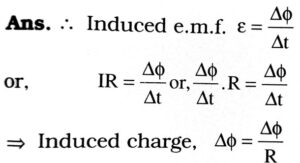It means Δφ does not depend upon the rate of change in magnetic flux.

Q.9. State Lenz’s law. The closed loop PQRS is moving into a uniform magnetic field acting at right angles to the plane of the paper. State the direction in which induced current flows in the loop.

Ans ⇒  Lenz’s law states that when there is the change in magnetic flux linked with the coil, an cause of its production.
The direction of induced current in the loop will be anticlockwise i.e., along SRQP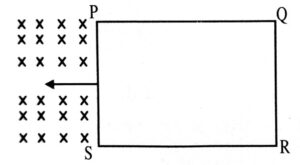Q.10. When a magnet is moved towards a suspended wire loop as shown in figure. Evaluate the direction of induced current in loop. State the law used by you for this evaluation.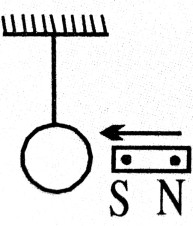Ans ⇒  When south pole of a magnet moves towards a suspended wire loop the direction of induced current is such that the surface of the wire loop facing south pole of the moving magnet behaves as south pole so that it may oppose the cause by which it is produced. This is an accordance with Lenz’s law.

Q.11. Write down S.I. unit and dimension of magnetic flux.

Ans ⇒  S.I. unit of magnetic flux is Tm2‘ which is called ‘Weber’.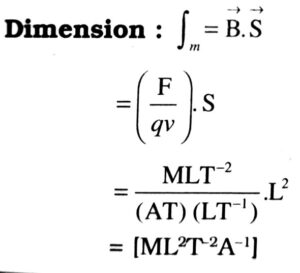## Class 12th physics Subjective question in English

 S.N Physics Short Type Question English Medium 1. ELECTRIC CHARGES AND FIELDS 2. LECTROSTATIC POTENTIAL AND CAPACITANCE 3. CURRENT ELECTRICITY 4. MOVING CHARGES AND MAGNETISM 5. MAGNETISM AND MATTER 6. ELECTROMAGNETIC INDUCTION 7. ALTERNATING CURRENT 8. ELECTROMAGNETIC WAVES 9. RAY OPTICS AND OPTICAL INSTRUMENTS 10. WAVE OPTICS 11. DUAL NATURE OF MATTER AND RADIATION 12. ATOMS 13. NUCLEI 14. SEMI CONDUCTOR ELECTRONICS 15. COMMUNICATION SYSTEMS